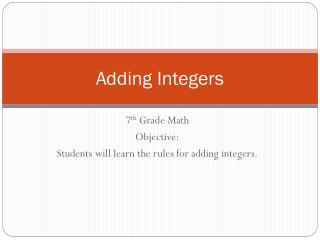DownloadDownload PresentationTélécharger la présentation- - - - - - - - - - - - - - - - - - - - - - - - - - - E N D - - - - - - - - - - - - - - - - - - - - - - - - - - -
##### Presentation Transcript

2. Vocabulary • Opposites • Two numbers who have the same absolute value, but different signs • Additive Inverse • Two numbers that when added together have a sum of zero • Also called “Zero Pairs”

3. Rule – Same Sign • When the signs are the same, add the numbers. • Keep the sign.

4. Same Sign • -6 + (-8) = • 4 + 5 = • -16 + (-16) + (-2) = -14 9 -34

5. Rule - Different Sign • When the signs are different, subtract the numbers. • Use the sign of the number with the greater absolute value.

6. Different Sign • -15 + 8 = • 7 + (-11) = • 25 + 3 + (-25) = -7 -4 3

7. Word Problems • Lena was scuba diving 14 meters below the surface of the water. She saw a nurse shark 3 meters above her. Write an addition expression to describe the situation. -14 + 3

8. Word Problems • Stephanie has \$152 in the bank. She withdrawals \$20 on Tuesday. She then deposits \$84 on Friday. Write an addition expression to describe the situation. 152 + (-20) + 84